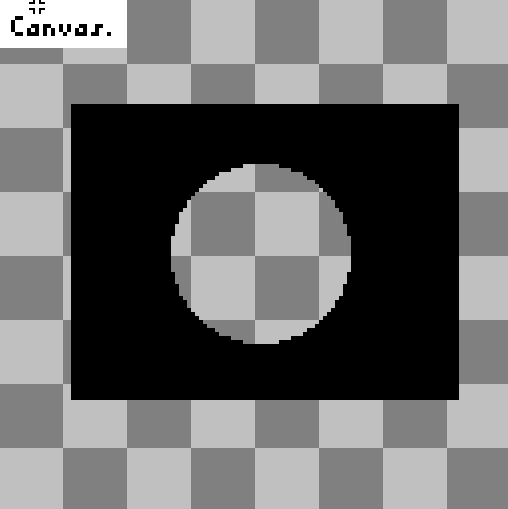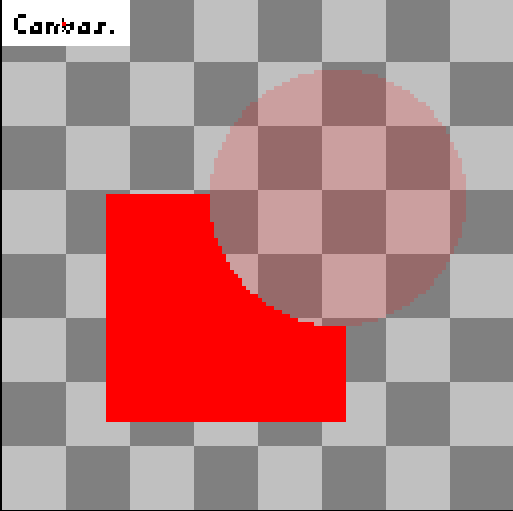That problem confuse me for a long time.
If I want to draw a rectangle with a hole manually like this:Grey grid is the transparent area
Here's the node code

``````extends Node2D
func _draw():
draw_rect(Rect2(50, 200, 150, 150), Color(1, 0, 0, 0))
draw_circle(Vector2(200, 200), 100, Color(1, 0, 0, 0.9))
``````

``````shader_type canvas_item;

void fragment() {
COLOR.a = 1.0 - COLOR.a;
}
``````